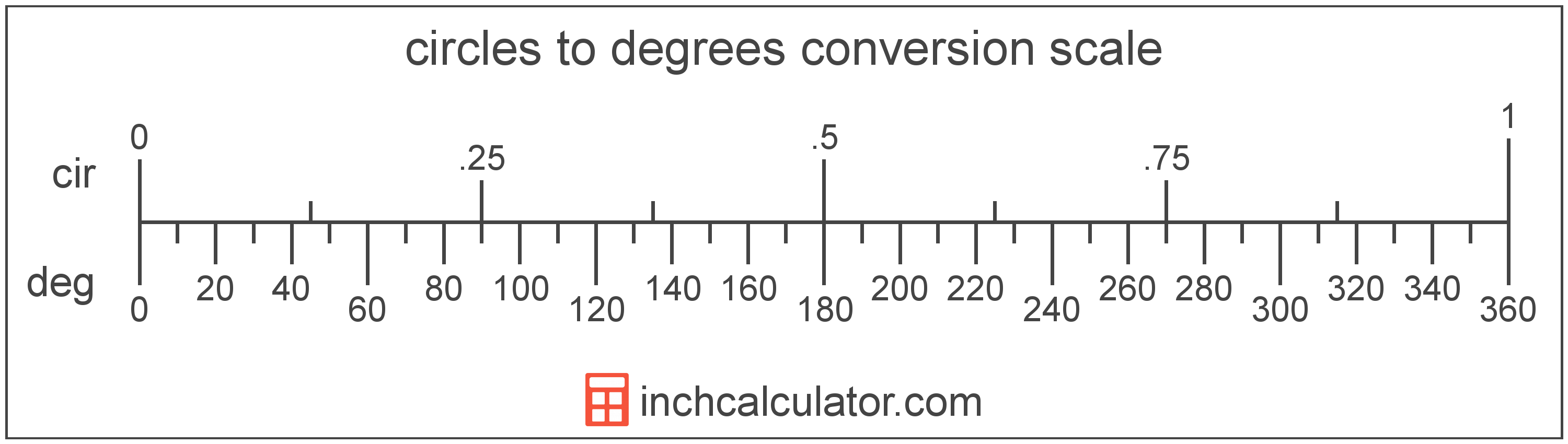# Degrees to Circles Conversion

Enter the angle in degrees below to get the value converted to circles.

Results in Circles:1° = 0.002778 cir
Do you want to convert circles to degrees?

## How to Convert Degrees to CirclesTo convert a degree measurement to a circle measurement, divide the angle by the conversion ratio.

Since one circle is equal to 360 degrees, you can use this simple formula to convert:

circles = degrees ÷ 360

The angle in circles is equal to the degrees divided by 360.

For example, here's how to convert 500 degrees to circles using the formula above.
500° = (500 ÷ 360) = 1.388889 cir

## Degrees

A degree is a measure of angle equal to 1/360th of a revolution, or circle. The number 360 has 24 divisors, making it a fairly easy number to work with. There are also 360 days in the Persian calendar year, and many theorize that early astronomers used 1 degree per day.

The degree is an SI accepted unit for angle for use with the metric system. A degree is sometimes also referred to as a degree of arc, arc degree, or arcdegree. Degrees can be abbreviated as °, and are also sometimes abbreviated as deg. For example, 1 degree can be written as 1° or 1 deg.

Degrees can also be expressed using minutes and seconds as an alternative to using the decimal form. Minutes and seconds are expressed using the prime (′) and double-prime (″) characters, although a single-quote and double-quote are often used for convenience.

One minute is equal to 1/60th of a degree, and one second is equal to 1/60th of a minute.

Protractors are commonly used to measure angles in degrees. They are semi-circle or full-circle devices with degree markings allowing a user to measure an angle in degrees. Learn more about how to use a protractor or download a printable protractor.

## Circles

A circle is the equivalent of 1 revolution around a circle, or 360°.

A circle is sometimes also referred to as a revolution. Circles can be abbreviated as cir; for example, 1 circle can be written as 1 cir.

## Degree to Circle Conversion Table

Degree measurements converted to circles
Degrees Circles
0.002778 cir
0.005556 cir
0.008333 cir
0.011111 cir
0.013889 cir
0.016667 cir
0.019444 cir
0.022222 cir
0.025 cir
10° 0.027778 cir
20° 0.055556 cir
30° 0.083333 cir
40° 0.111111 cir
50° 0.138889 cir
60° 0.166667 cir
70° 0.194444 cir
80° 0.222222 cir
90° 0.25 cir
100° 0.277778 cir
200° 0.555556 cir
300° 0.833333 cir
400° 1.1111 cir
500° 1.3889 cir
600° 1.6667 cir
700° 1.9444 cir
800° 2.2222 cir
900° 2.5 cir
1,000° 2.7778 cir

## References

1. Collins Dictionary, Definition of 'degree', https://www.collinsdictionary.com/us/dictionary/english/degree